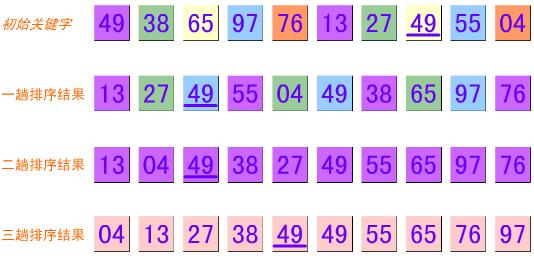# 波斯马BOSSMA Information Technology

## C#排序算法之希尔排序（shellsort）

49,38,65,97,76,13,27,49,55,04

5,3,1```/// <summary>
/// 希尔排序
/// </summary>
static void ShellSort(int[] intArr)
{
int numberOfLoop = 0;
int numberOfSwap = 0;

//每组数量，即增量
int numberOfPerGroup;

//获取初始增量值
//关于最优增量值如何得出，没有找到可用的资料，这里参考网上常见的数值
for (numberOfPerGroup = 1; numberOfPerGroup <= intArr.Length / 9; numberOfPerGroup = 3 * numberOfPerGroup + 1) ;

//增量递减
for (; numberOfPerGroup > 0; numberOfPerGroup = numberOfPerGroup / 3)
{
numberOfLoop++;

//这里就是直接插入排序了
for (int i = 0; i < intArr.Length; i += numberOfPerGroup)
{
int j;
int temp = intArr[i];

for (j = i; j >= numberOfPerGroup; j -= numberOfPerGroup)
{
numberOfLoop++;

if (intArr[j - numberOfPerGroup] > temp)
{
numberOfSwap++;
intArr[j] = intArr[j - numberOfPerGroup];
}
else
{
break;
}
}

numberOfLoop++;
intArr[j] = temp;
}
}
```

【上一篇】 【下一篇】

### 发表评论

##### 相关文章